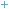International Baccalaureate Middle Years Programme

Summary of the Math Criteria:

 Criterion A Knowledge and understanding Maximum 8 Criterion B Investigating patterns Maximum 6* Criterion C Communication in mathematics Maximum 6 Criterion D Reflection in mathematics Maximum 6

Detailed Descriptors:

### Criterion A: knowledge and understanding

Maximum 8

Knowledge and understanding are fundamental to studying mathematics and form the base from which to explore concepts and develop skills. This criterion expects students to use their knowledge and to demonstrate their understanding of the concepts and skills of the prescribed framework in order to make deductions and solve problems in different situations, including those in real-life contexts.

This criterion examines to what extent the student is able to:

·          know and demonstrate understanding of the concepts from the five branches of mathematics (number, algebra, geometry and trigonometry, statistics and probability, and discrete mathematics)

·          use appropriate mathematical concepts and skills to solve problems in both familiar and unfamiliar situations, including those in real-life contexts

·          select and apply general rules correctly to solve problems, including those in real-life contexts.

 Achievement level Descriptor 0 The student does not reach a standard described by any of the descriptors given below. 1–2 The student attempts to make deductions when solving simple problems in familiar contexts. 3–4 The student sometimes makes appropriate deductions when solving simple and more-complex problems in familiar contexts. 5–6 The student generally makes appropriate deductions when solving challenging problems in a variety of familiar contexts. 7–8 The student consistently makes appropriate deductions when solving challenging problems in a variety of contexts including unfamiliar situations.

Notes

1.       Context: the situation and the parameters given to a problem.

2.       Unfamiliar situation: challenging questions or instructions set in a new context in which students are required to apply knowledge and/or skills they have been taught.

3.       Deduction: reasoning from the general to the particular/specific.

### Criterion B: investigating patterns

Maximum 6

Students are expected to investigate a problem by applying mathematical problem-solving techniques, to find patterns, and to describe these mathematically as relationships or general rules and justify or prove them.

This criterion examines to what extent the student is able to:

·          select and apply appropriate inquiry and mathematical problem-solving techniques

·          recognize patterns

·          describe patterns as relationships or general rules

·          draw conclusions consistent with findings

 Achievement level Descriptor 0 The student does not reach a standard described by any of the descriptors given below. 1–2 The student applies, with some guidance, mathematical problem-solving techniques to recognize simple patterns. 3–4 The student selects and applies mathematical problem-solving techniques to recognize patterns, and suggests relationships or general rules. 5–6 The student selects and applies mathematical problem-solving techniques to recognize patterns, describes them as relationships or general rules, and draws conclusions consistent with findings.

Notes

1.       Pattern: the underlining order, regularity or predictability between the elements of a mathematical system. To identify pattern is to begin to understand how mathematics applies to the world in which we live. The repetitive features of patterns can be identified and described as relationships or generalized rules.

### Criterion C: communication in mathematics

Maximum 6

Students are expected to use mathematical language when communicating mathematical ideas, reasoning and findings—both orally and in writing.

This criterion examines to what extent the student is able to:

·          use appropriate mathematical language (notation, symbols, terminology) in both oral and written explanations

·          use different forms of mathematical representation (formulae, diagrams, tables, charts, graphs and models)

·          move between different forms of representation.

Students are encouraged to choose and use appropriate ICT tools such as graphic display calculators, screenshots, graphing, spreadsheets, databases, drawing and word-processing software, as appropriate, to enhance communication.

 Achievement level Descriptor 0 The student does not reach a standard described by any of the descriptors given below. 1–2 The student shows basic use of mathematical language and/or forms of mathematical representation. The lines of reasoning are difficult to follow. 3–4 The student shows sufficient use of mathematical language and forms of mathematical representation. The lines of reasoning are clear though not always logical or complete. The student moves between different forms of representation with some success. 5–6 The student shows good use of mathematical language and forms of mathematical representation. The lines of reasoning are concise, logical and complete. The student moves effectively between different forms of representation.

Notes

1.       Mathematical language: the use of notation, symbols, terminology and verbal explanations.

2.       Forms of mathematical representation: refers to formulae, diagrams, tables, charts, graphs and models, used to represent mathematical information.

### Criterion D: reflection in mathematics

Maximum 6

Reflection allows students to reflect upon their methods and findings.

This criterion examines to what extent the student is able to:

·          explain whether his or her results make sense in the context of the problem

·          explain the importance of his or her findings in connection to real life

·          justify the degree of accuracy of his or her results where appropriate

·          suggest improvements to the method when necessary.

 Achievement level Descriptor 0 The student does not reach a standard described by any of the descriptors given below. 1–2 The student attempts to explain whether his or her results make sense in the context of the problem. The student attempts to describe the importance of his or her findings in connection to real life. 3–4 The student correctly but briefly explains whether his or her results make sense in the context of the problem and describes the importance of his or her findings in connection to real life. The student attempts to justify the degree of accuracy of his or her results where appropriate. 5–6 The student critically explains whether his or her results make sense in the context of the problem and provides a detailed explanation of the importance of his or her findings in connection to real life. The student justifies the degree of accuracy of his or her results where appropriate. The student suggests improvements to the method when necessary.MYP Course IndexMYP InformationDP InformationIBO website